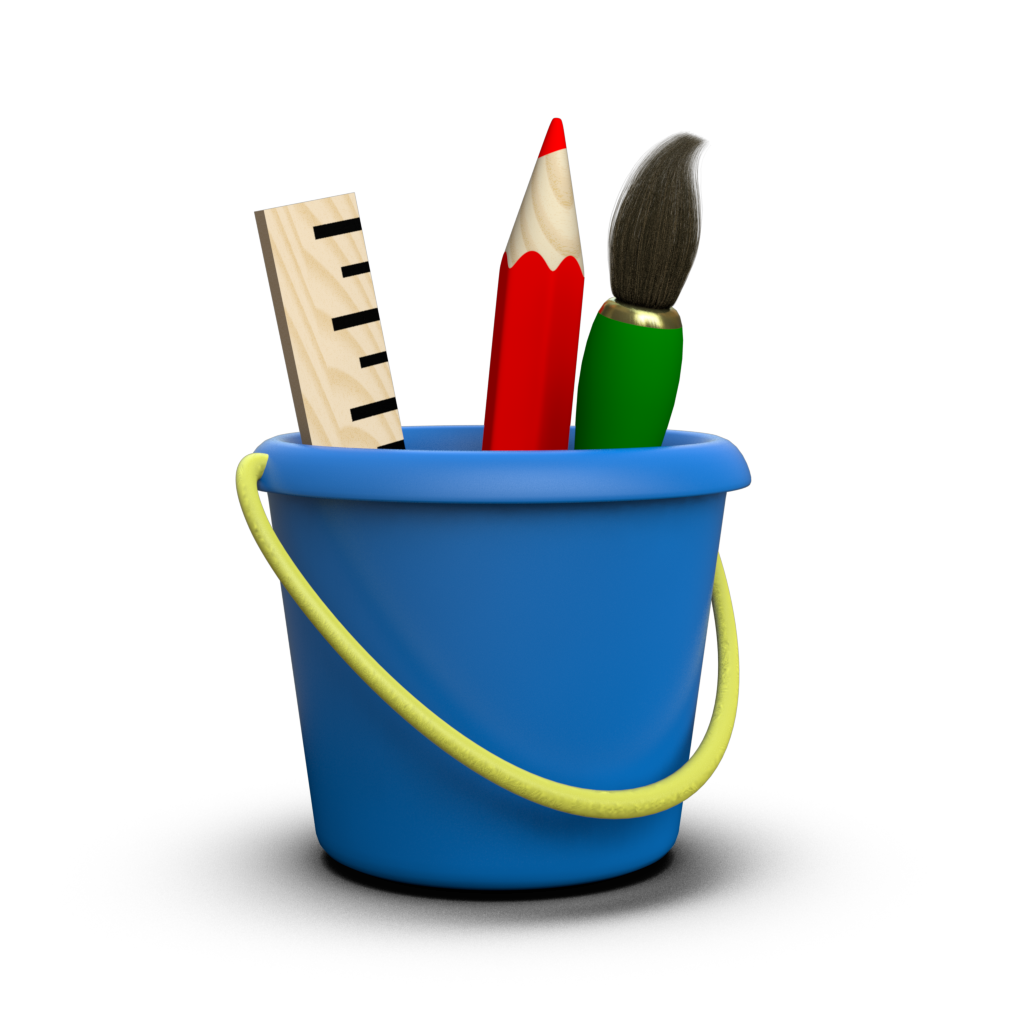# Ellipse Visualization¶

Toyplot supports drawing oriented ellipses using toyplot.coordinates.Cartesian.ellipse(). Ellipses are defined using their center, x and y radius, and angle with respect to the X axis. For example, to create an unrotated ellipse centered at the origin:

:

import toyplot

canvas = toyplot.Canvas(width=400)
axes = canvas.cartesian(aspect="fit-range")
axes.ellipse(0, 0, 5, 2);


When rotating ellipses, angles are specified in degrees with positive angles leading to clockwise rotation:

:

canvas = toyplot.Canvas(width=400)
axes = canvas.cartesian(aspect="fit-range")
axes.ellipse(0, 0, 5, 2, 45);


Of course, you can use color, opacity, and style to alter the appearance of an ellipse:

:

canvas = toyplot.Canvas(width=600, height=300)
axes = canvas.cartesian(grid=(1, 2, 0), aspect="fit-range")
axes.ellipse(0, 0, 5, 2, 45, color="crimson", opacity=0.5)
axes = canvas.cartesian(grid=(1, 2, 1), aspect="fit-range")
axes.ellipse(0, 0, 5, 2, 45, style={"stroke": "black", "fill":"none"});


One thing to keep in mind when working with ellipses is that, if you don’t specify an explicit domain for your axes, Toyplot’s default axis scaling will tend to turn an ellipse into a circle:

:

canvas = toyplot.Canvas(width=300)
axes = canvas.cartesian() # Allow Toyplot to choose the domain
axes.ellipse(0, 0, 5, 2);


This is because Toyplot treats your ellipses as data - that is, ellipses aren’t just symbols drawn atop the canvas, they are embedded in the space defined by the axes. Thus, an ellipse will be distorted when viewed using a nonlinear projection such as log axes. This is by design:

:

canvas = toyplot.Canvas(width=600, height=300)
axes = canvas.cartesian(grid=(1, 2, 0), label="Linear Y projection")
axes.ellipse(20, 15, 20, 5, 30)
axes = canvas.cartesian(grid=(1, 2, 1), yscale="log", label="Nonlinear Y projection")
axes.ellipse(20, 15, 20, 5, 30);


The distortion can become even more extreme if the ellipse straddles the origin:

:

canvas = toyplot.Canvas(width=600, height=300)
axes = canvas.cartesian(grid=(1, 2, 0), label="Linear Y projection")
axes.ellipse(0, 0, 20, 5, 30)
axes = canvas.cartesian(grid=(1, 2, 1), yscale="log", label="Nonlinear Y projection")
axes.ellipse(0, 0, 20, 5, 30);


While this behavior may seem nonintuitive at first, it makes sense when you’re using an ellipse to visualize confidence or other bounds for sampled data. For example, suppose you’re drawing samples from a bivariate normal distribution:

:

import numpy
numpy.random.seed(1234)

mean = numpy.array([3, 5])
covariance = numpy.array([[3, 1], [1, 1]])

samples = numpy.random.multivariate_normal(mean, covariance, size=1000)

canvas = toyplot.Canvas()
axes = canvas.cartesian(aspect="fit-range")
axes.scatterplot(samples[:,0], samples[:,1]);


If you wanted to draw ellipses at 1, 2, and 3-$$\sigma$$ intervals, you might do the following:

:

eigenvalues, eigenvectors = numpy.linalg.eig(covariance)
angle = numpy.degrees(numpy.arctan2(eigenvectors[1, 0], eigenvectors[0, 0]))

xsigma = numpy.sqrt(eigenvalues)
ysigma = numpy.sqrt(eigenvalues)

canvas = toyplot.Canvas()
axes = canvas.cartesian(aspect="fit-range")
axes.scatterplot(samples[:,0], samples[:,1])
axes.ellipse(mean, mean, xsigma, ysigma, angle, style={"stroke":"black", "fill":"none"})
axes.ellipse(mean, mean, xsigma * 2, ysigma * 2, angle, style={"stroke":"black", "fill":"none"})
axes.ellipse(mean, mean, xsigma * 3, ysigma * 3, angle, style={"stroke":"black", "fill":"none"});


However, if you use a nonlinear projection on the Y axis, the ellipse needs to deform along with the rest of the data:

:

canvas = toyplot.Canvas(width=600, height=400)
axes = canvas.cartesian(aspect="fit-range", yscale="log")
axes.scatterplot(samples[:,0], samples[:,1])
axes.ellipse(mean, mean, xsigma, ysigma, angle, style={"stroke":"black", "fill":"none"})
axes.ellipse(mean, mean, xsigma * 2, ysigma * 2, angle, style={"stroke":"black", "fill":"none"})
axes.ellipse(mean, mean, xsigma * 3, ysigma * 3, angle, style={"stroke":"black", "fill":"none"});


Finally, as with other Toyplot visualizations, you are not limited to creating individual ellipses one-at-a-time - you can create a series of ellipses in a single call:

:

numpy.random.seed(14)

x = numpy.random.uniform(-6, 6, size=10)
y = numpy.random.uniform(-4, 4, size=10)
rx = numpy.random.uniform(0.1, 1, size=10)
ry = numpy.random.uniform(0.1, 1, size=10)
angle = numpy.random.uniform(0, 180, size=10)

canvas = toyplot.Canvas(width=600, height=400)
axes = canvas.cartesian(aspect="fit-range")
axes.ellipse(x, y, rx, ry, angle, color=toyplot.color.brewer.map("Set1"), style={"stroke":"white"});